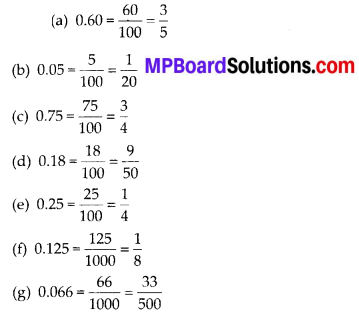# MP Board Class 6th Maths Solutions Chapter 8 Decimals Ex 8.2

## MP Board Class 6th Maths Solutions Chapter 8 Decimals Ex 8.2

Question 1.
Complete the table with the help of these boxes and use decimals to write the number,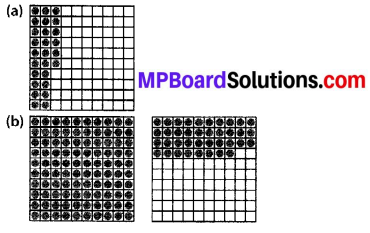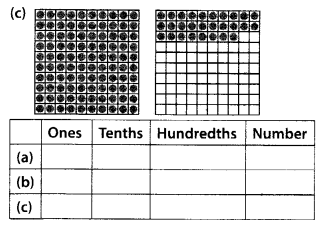Solution: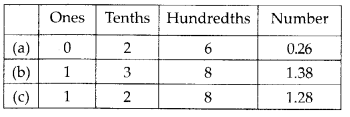Question 2.
Write the numbers given in the following place value table in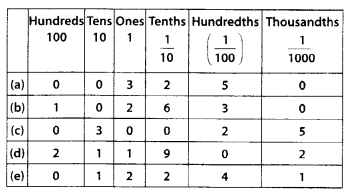Solution: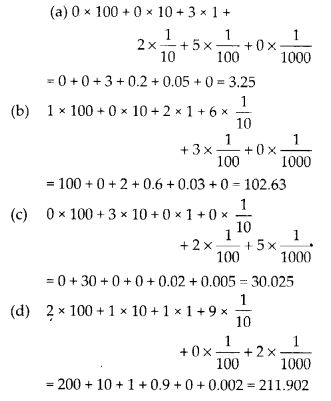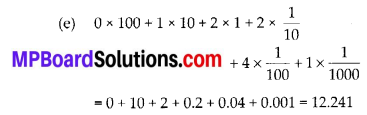Question 3.
Write the following decimals in the place value table.
(a) 0.29
(b) 2.08
(c) 19.60
(d) 148.32
(e) 200.812
Solution: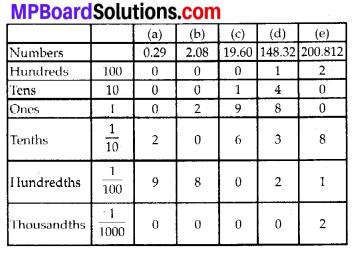Question 4.
Write each of the following as decimals.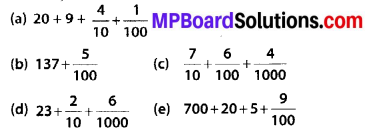Solution:
(a) 20 + 9 + 0.4 + 0.01 = 29.41
(b) 137 + 0.05 = 137.05
(c) 23 + 0.2 + 0.006 = 23.206
(d) 700 + 20 + 5 + 0.09 = 725.09Question 5.
Write each of the following decimals in words.
(a) 0.03
(b) 1.20
(c) 108.56
(d) 10.07
(e) 0.032
(f) 5.008
Solution:
(a) Zero point zero three
(b) One point two zero
(c) One hundred and eight point five six
(d) Ten point zero seven
(e) Zero point zero three two
(f) Five point zero zero eightQuestion 6.
Between which two numbers in tenths place on the number line does each of the given number lie?
(a) 0.06
(b) 0.45
(c) 0.19
(d) 0.66
(e) 0.92
(f) 0.57
Solution:
(a) 0.06 lies between 0 and 0.1.
(b) 0.45 lies between 0.4 and 0.5.
(c) 0.19 lies between 0.1 and 0.2.
(d) 0.66 lies between 0.6 and 0.7.
(e) 0.92 lies between 0.9 and 1.0.
(f) 0.57 lies between 0.5 and 0.6.

Question 7.
Write as fractions in lowest terms.
(a) 0.60
(b) 0.05
(c) 0.75
(d) 0.18
(e) 0.25
(f) 0.125
(g) 0.066
Solution: